Home > CC1 > Chapter 8 > Lesson 8.1.4 > Problem8-55

8-55.
1. Use the Distributive Property to rewrite each expression below. Then evaluate each expression for y = 3 and z = 4. Homework Help ✎

1. 2y2 − 5y

2. 3(8 − 2z)

3. 5z2 −15z

4. 4y + 6z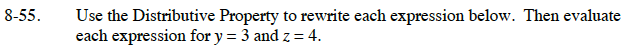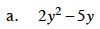Think of this expression as (y · 2y) − (y · 5).

Using the Distributive Property, the expression becomes
y(2y − 5). When 3 is substituted for y, the expression becomes 3(2 · 3 − 5) = 3(6 − 5) = 3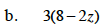Think of the expression as 3 · 8 − 3 · 2z.

The expression becomes 24 − 6z. Now substitute 4 for z.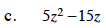Both numbers and variables can be factored out together. Solve this one like you did part (a).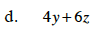Be careful with the variables. Remember that because the variables are different, they cannot be factored out. However, there is a number that can be factored out.